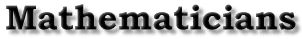Life | Accomplishments | Pascal's Theorem | Bibliography | Back to the front page Pascal's Theorem Julia Chew Pascal's favorite mathematical topic to study, geometry, led to the formulation of Pascal's theorem. This states that pairs of opposite sides of a hexagon inscribed in any conic section meet in three collinear points. Pascal published this as Essai pour les Coniques when he was just sixteen years old. He had planned to publish a complete treatise on the subject but somehow, it was never published. All that remains on his work with conics is the essay, which is the introduction to the larger piece. Unfortunately, only a few copies were ever published of which only two remain, one in the Bibliothèque Nationale in Paris and one in the Leibniz collection in Hanover, Germany. (Hazelton) Pascal's interest in conic sections most likely came about from his love of geometry and his association with Desargues, who was a great contributor to the study of conics. In his Essai, Pascal expresses his gratitude to the teachings and writings of Desargues. Pascal's study of conics follows from the theory of Desargues. He uses many of the theorems introduced in Desargues writings. The generation of conic sections is described by Pascal in the first portion of his work. A cone is constructed with a circle, a fixed point in space not in the plane of the circle, and a straight line through the point and the circumference of the circle. The line moves around the circumference of the circle, generating a conic surface. (Davidson) The surface of the two cones generated stretch out to infinity. Next, Pascal introduces a plane that also extends infinitely. Thus the two figures will meet with the plane cutting the cone. It can do this in six different ways producing six different sections: a point, a straight line, an angle, a closed figure (a circle or ellipse), a parabola, or a hyperbola. (Davidson) Next Pascal describes the properties of what he calls the hexagramme mystique. Every conic section corresponds to a hexagon. Pascal's famous theorem, also known as the Mystic Hexagram, states: If any six sided, six angled figure is inscribed in any conic section, and the sides of the hexagon thus produced are projected beyond the section, the pairs of opposite sides will meet in three points all of which lie on a straight line. (Hazelton) Take any conic section, say a circle. Then inscribe a hexagon inside the circle. Next extend the sides of the hexagon. The three points p,q,r where the pairs of opposite sides meet lie on a straight line. (see illustration 1) Alternately, one can also inscribe a hexagon in a circle and draw the lines from each vertex to the two opposite vertices. The points P,Q,R where the lines meet lie in a straight line. (see illus. 2) Pascal never proved this theorem. He simply stated that the results follow from Desargues work. (Katz) He completed his Treatise on conics, but why it was never published is not known. After his death, his nephew sent the Treatise to Leibniz to examine and deem whether or not it was in a state that could be published. After the papers were returned, they disappeared. All that is known of this work is what is told by Leibniz. He copied the introduction of the Treatise, which was published as the Essai. Leibniz categorized Pascal's treatise into six sections. The second section further discusses the mystic hexagram and its properties. The third discusses tangents and lines joining their intersecting points. The fourth deals with secants and tangents. The fifth concerns the determination of conic sections passing through given points or conics tangent to given lines. The last section deals with Pappus's problem. (Davidson) This problem states: Given three or four straight lines, to find the geometrical locus of points such that, if one draws from the points rectilinear segments cutting the straight lines at given angles, the product of two of these segments will be equal to the third or to the product of the third by the fourth. (Bishop) Life | Accomplishments | Pascal's Theorem | Bibliography | Back to the front page